Courses

# Centre Of Mass NAT Level - 1

## 10 Questions MCQ Test Topic wise Tests for IIT JAM Physics | Centre Of Mass NAT Level - 1

Description
This mock test of Centre Of Mass NAT Level - 1 for IIT JAM helps you for every IIT JAM entrance exam. This contains 10 Multiple Choice Questions for IIT JAM Centre Of Mass NAT Level - 1 (mcq) to study with solutions a complete question bank. The solved questions answers in this Centre Of Mass NAT Level - 1 quiz give you a good mix of easy questions and tough questions. IIT JAM students definitely take this Centre Of Mass NAT Level - 1 exercise for a better result in the exam. You can find other Centre Of Mass NAT Level - 1 extra questions, long questions & short questions for IIT JAM on EduRev as well by searching above.
*Answer can only contain numeric values
QUESTION: 1

### A block of mass 0.50 kg is moving with a speed of 2.00 ms–1 on a smooth surface. It strikes another mass of 1.00 kg and then they move together as a single body. The energy loss (in Joule) during the collision is :

Solution:

pi = pf  (momentum conservation)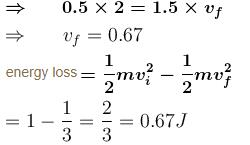*Answer can only contain numeric values
QUESTION: 2

### A force time graph for a linear motion is shown in figure where the segments are circular. The linear momentum gained between 0 to 8 second is :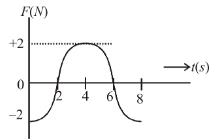Solution:

Change in momentum = area F-t graph = 0

*Answer can only contain numeric values
QUESTION: 3

### A bullet of mass  m = 50gm strikes (Δt ≈ 0) a sand bag of mass M = 5 kg hanging from a fixed point with a horizontal velocity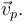If bullet sticks to the sand bag then the ratio of final and initial kinetic energy of the bullet is 10-α. Find the value of α:

Solution: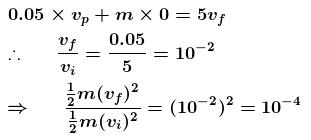*Answer can only contain numeric values
QUESTION: 4

A shell of mass 100 kg is fired horizontally from a canon with a mass of 5 × 103kg. The kinetic energy of the shell at the end of the barrel is 7.5 × 106 J. The kinetic energy imparted to the cannon by the recoil is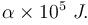Find the value of  α:

Solution:

m = 100 kg
M = 5×103 kg
Initially system is at rest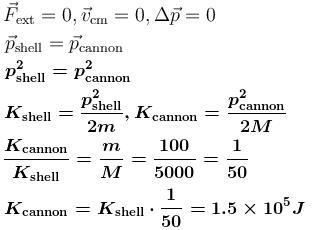*Answer can only contain numeric values
QUESTION: 5

Two masses of 1gm and 4gm are moving with equal kinetic energy. Find the sum of the magnitude of their linear momentum :

Solution:

KE= 1/2 m*v^2

Momentum (p)=m*v

Set each of the 1 gram and 4 grams KE to 8:

KE=1/2 *1* (4)^2=8

KE=1/2 *4* (2)^2=8

The 1 gram takes 4 m/s to achieve KE of 8.

The 4 gram takes 2 m/s to achieve KE of 8.

Therefore, the ratio of their linear momentum (m*v) is:

1*4/4*2= 4/8= 1/2

*Answer can only contain numeric values
QUESTION: 6

A ball of mass 50gm is dropped from a height = 10m. It rebounds losing 75 percent of its kinetic energy. If it remains in contact with the ground for  Δt = 0.01 sec., the impulse of the impact force (in N-s)  is : (take =10 m/s2)

Solution: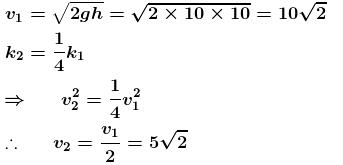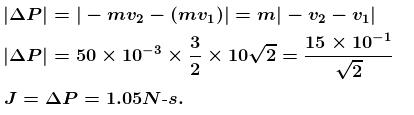*Answer can only contain numeric values
QUESTION: 7

A ball collides with an inclined plane of inclination θ after falling through a distance h. If it moves horizontally just after the impact, the coefficient of restitution is, when θ = 30: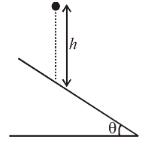Solution: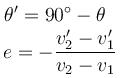where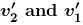are velocities components of wedge and ball along the impulsive force after collision and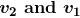are velocities components of wedge and ball along the impulsive force before collision
Wedge is at rest so,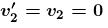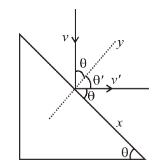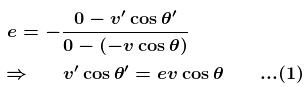conserving the linear momentum in x-direction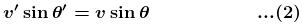On dividing equation (2) by (1)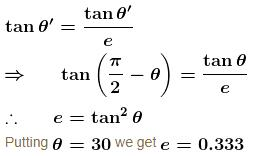*Answer can only contain numeric values
QUESTION: 8

A body of mass = 3.513 kg is moving along the x-axis with a speed of  5.00 m/s–1. The magnitude of its momentum (in kgms –1) is recorded as :

Solution: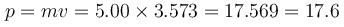As the lowest no. of significant figure is 3.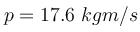*Answer can only contain numeric values
QUESTION: 9

Three particles of mass 20gm, 30gm and 40gm are initially moving along the positive direction of the three coordinate axes respectively with the same velocity of 20 cm/s. If due to their mutual interaction, the first particle comes to rest, the second acquires a velocity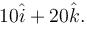What is the magnitude of velocity (in cm/s) of the third particle?

Solution: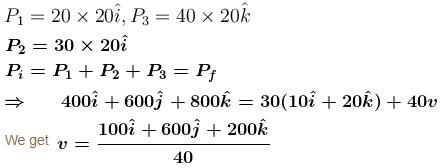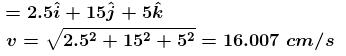*Answer can only contain numeric values
QUESTION: 10

Two particles of mass 1kg and 0.5kg are moving in the same direction with speed of 2 m/s and 6 m/s respectively on a smooth horizontal surface. The speed of centre of mass of the system in m/s is :

Solution: